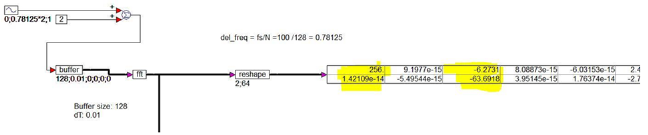## !=Block Category: Boolean

Inputs: Real, complex, or fixed-point scalars, or vectors or matrices.

l: numerator x1.

r: denominator x2.

Description: The != block generates an output signal of 1 if and only if the two scalar input signals are not equal.

Right-click the != block, to assign a different function to the block.

#### Example

1. Comparing constants

Consider a variable y such that:

If t ≠ 0.5 then y = cos(t); else y = 0

where t is simulation time realized as:Until the value of t reaches 0.5, the Boolean inequality t 0.5 evaluates to true, and y takes on a value of cos(t). At t = 0.5 sec, the Boolean inequality evaluates to false, and at the very next time step, returns to true, and remains true for the duration of the simulation. Consequently, at the moment t = 0.5 sec, y takes on the value of 0, and at every other point, y is equal to cos(t).